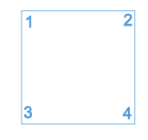SSAT Elementary Level Math : How to find the perimeter of a square

Example Questions

1 2 4 Next →

Example Question #31 : How To Find The Perimeter Of A Square

Find the perimeter of a square with side length 4.Explanation:

To solve, simply use the formula for the perimeter of a square. Thus,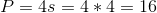You can also solve this by simply adding up all of the sides of a square.

What is the area of a square with a perimeter of 12 cm?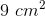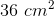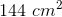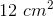Explanation:

Based on the fact that a Square has four equal sides, we must first divide 12 by 4 to find the measure of each side.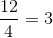All of the sides are 3 cm in length.

Now to find the area of a square we must multiply the base and the height or take one side and square it. In both cases we get the below equation: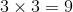This gives us the answer which is:Example Question #51 : Squares

Use the following to answer the question.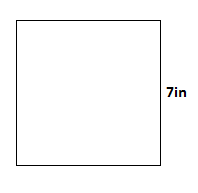Find the perimeter of the square.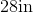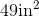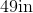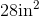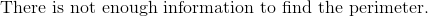Explanation:

To find the perimeter of a square, we will use the following formula: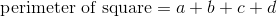where a, b, c, and d are the lengths of the sides of the square.

If we look at the squarewe can see one length is 7in.  Because it is a square, we know that all of the sides are equal or the same.  Therefore, all sides are 7in.  Knowing this, we can substitute into the formula.  We get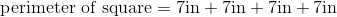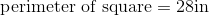Example Question #52 : Plane Geometry

Find the perimeter of the given square.

What is the perimeter of the square if one side of the square is 24cm.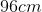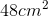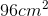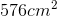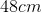Explanation:

To find the perimeter of a square, you must first understand that all sides of a square are equal.  To find the perimeter of a figure you add all sides together. To find the perimeter of this square you could use two ways.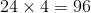or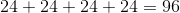Either way you will end with the same answer 96cm.  The cm are not squared when finding perimeter because you are adding your values together, not multiplying them.

Find the perimeter of the square.

The square has a side of 4.5in. What is the perimeter of the square.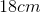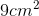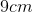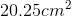Explanation:

First you must understand that a square has 4 equal sides. So to find the perimeter of that square you can take one side and add that four times or multiply that side by 4.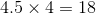or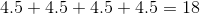Therefore you answer would be 18cm. The cm are not squared as the sides are added together, not multiplied.

Use the following square to answer the question: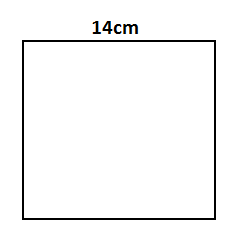Find the perimeter.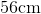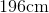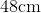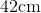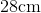Explanation:

To find the perimeter of a square, we will use the following formula:where a, b, c, and d are the lengths of the sides of the square.

Now, let's look at the square:We can see it has a length of 14cm.  Because it is a square, all sides are equal.  Therefore, all sides are 14cm.

Knowing this, we can substitute into the formula.  We get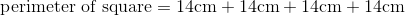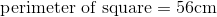Use the following square to solve the problem: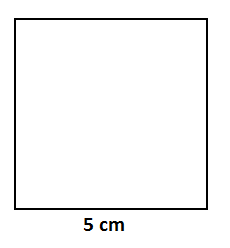Find the perimeter.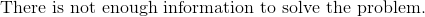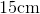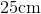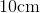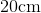Explanation:

To find the perimeter of a square, we will use the following formula: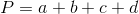where a, b, c, and d are the lengths of the sides of the square.

Now, given the squarewe can see the length is 5cm.  Because it is a square, all sides are equal.  Therefore, all sides are 5cm.  So, we will substitute.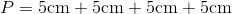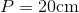The perimeter of a square is 24in.  Find the length of one side.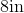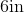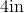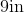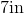Explanation:

To find the perimeter of a square, we can use the following formula: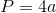where a is the length of one side.  Because a square has 4 equal sides, we can take 4 times the length of one side.  So, to find the length of one side, we will solve for a.

We know the perimeter of the square is 24in.  So, we will substitute.  We get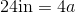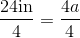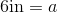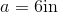Therefore, the length of one side of the square is 6in.

Example Question #2 : Recognize And Draw Shapes: Ccss.Math.Content.2.G.A.1

Which shape has onlyangles?

Cube

Hexagon

Pentagon

Triangle

Square

A square as onlyangles.